Custom SearchPROBABILITY OF SUCCESS If a trial must result in any of n equally likely ways, and if s is the number of successful ways and f is the number of failing ways, the probability of success iswhere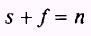EXAMPLE: What is the probability that a coin will land heads up? SOLUTION: There is only one way the coin can land heads up; therefore, s equals 1. There is also only one way the coin can land other than heads up; therefore, f equals 1. Since and f=1 then the probability of success is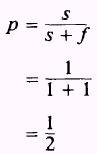This, then, is the ratio of successful ways in which the trial can succeed to the total number of ways the trial can result. EXAMPLE: What is the probability that a die (singular of dice) will land with a 3 showing on the upper face? SOLUTION: A die has a total of 6 sides. Therefore the die can land with a 3 face up 1 favorable way and 5 unfavorable ways. Since s=1 and f=5 thenEXAMPLE: What is the probability of drawing a black marble from a box of marbles if all 6 of the marbles in the box are white? SOLUTION: There are no favorable ways of success and there are 6 total ways. Therefore, s=0 and f=6 so that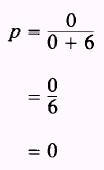EXAMPLE: What is the probability of drawing a black marble from a box of 6 black marbles? SOLUTION: There are 6 successful ways and no unsuccessful ways of drawing the marble. Therefore, s=6 and f=0 so thatThe previous two examples are the extremes of probabilities and intuitively demonstrate that the probability of an event ranges from 0 to 1 inclusively. EXAMPLE: A box contains 6 hard lead pencils and 12 soft lead pencils. What is the probability of drawing a soft lead pencil from the box? SOLUTION: We are given s=12 and f=6 therefore,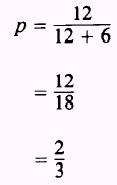PRACTICE PROBLEMS: 1. What is the probability of drawing an ace from a standard deck of 52 playing cards? 2. What is the probability of drawing a black ace from a standard deck of playing cards? 3. If a die is rolled, what is the probability of an odd number showing on the upper face? 4. A man has 3 nickels, 2 dimes, and 4 quarters in his pocket. If he draws a single coin from his pocket, what is the probability that a. he will draw a nickel? b. he will draw a half-dollar? c. he will draw a quarter? ANSWERS: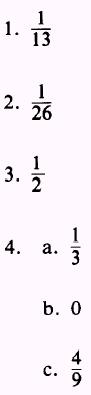Integrated Publishing, Inc. - A (SDVOSB) Service Disabled Veteran Owned Small Business